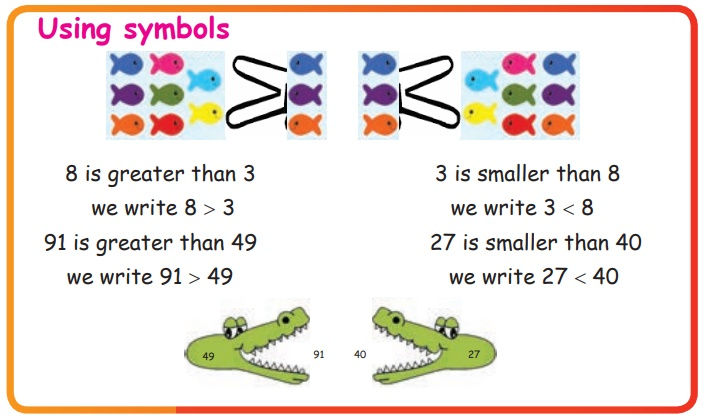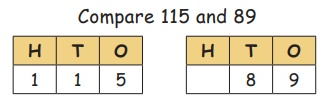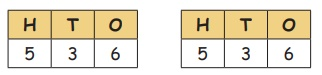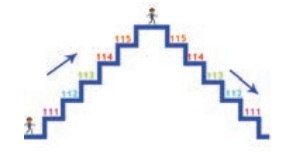Home | | Maths 3rd Std | Comparison of numbers

# Comparison of numbers

1. Odd and Even numbers 2. Greater and smaller numbers 3. Ascending and Descending order

Comparison of numbers

Odd and Even numbers

Ramu has 125 groundnut chocolates and Geetha has 200 groundnut chocolates. Who has more?Odd numbers and even numbersEven numbers 0 2 4 6 8 10 12

Odd numbers 1 3 5 7 9 11 13

Numbers ending with 1, 3, 5, 7 and 9 are called ODD numbers.

Numbers ending with 0, 2, 4, 6 and 8 are called EVEN numbers.

Activity 5

Colour the balloons with odd number by yellow and even number by Red.In number sequence, after every odd number there is an even number. Similarly after every even number there is an odd number.

Activity 6

Circle/Underline the even numbers

8, 69, 70, 84, 99

112, 131, 156, 170, 186

226, 300, 303, 440, 478

542, 570, 575, 600, 610

931, 948, 952, 982, 999

Circle/Underline the odd numbers

7, 26, 33, 61, 84

105, 116, 125, 142, 151

219, 232, 245, 357, 390

540, 555, 557, 603, 609

918, 919, 935, 953, 998

Greater and smaller numbers

Amuthan has 3 chocolates and his sister Meenakshi has 8 chocolates.Who has more?

In a number line 3 comes before 8 or 8 comes after 3.3 is smaller than 8 or

8 is greater than 3

Meenakshi has more chocolates.

Know more: ‘0’ does not have any value at the beginning of a number.

Using symbols8 is greater than 3

we write 8 > 3

91 is greater than 49

we write 91 > 49

3 is smaller than 8

we write 3 < 8

27 is smaller than 40

we write 27 < 40

1. Comparison of numbers with different digits.

The number which has more digits is a greater number. The number 115 has 3 digits and 89 has only 2 digits. So 115 is greater than 89. We write 115 > 89.

Compare 115 and 892. Comparison of numbers with equal digits.

If the number of digits are equal, compare the digit in the hundreds place. The number which has a greater digit in the hundreds place is greater. 2 is greater than 1. So, 250 is greater than 160. We write 250 > 160. We can also say 160 < 250.

Compare 160 and 250Look at the hundreds place

3. If the digits in the hundreds place are same, compare the digits in the tens place. The number which has the greater digit in the tens place is the greater number.

The digit in the hundred place are the same. Compare the digits in the tens place. 5 is greater than 4. So, 151 is greater than 143. We write 151 > 143. We can also say 143 < 151.4. If the digits in the hundred and the tens place are same, compare the digits in the ones place. The number which has the greater digit in the ones place is the greater number.

The digits in the hundred place and tens place the same.

Comparing the digits in the ones place.

Compare 141 and 1488 is greater than 1

So the number 148 is greater than 141.

We write 148 > 141

We can also say 141 < 148.

5. Comparing numbers with same value in all the digits

The digits in the hundreds places, tens place and ones place are same.

So, 536 = 536Try this

Put <, >, and = in the boxes provided.

103 __ 438 Answer: 103 < 438

250 __ 069 Answer: 250 > 069

408 __ 308 Answer: 408 > 308

710 __ 710 Answer: 710 = 710

614 __ 618 Answer: 614 < 618

719 __ 917 Answer: 719 < 917

The greatest three digit number is 999.

The smallest three digit number is 100.

Ascending and Descending order.

111, 112, 113, 114, 115

When we write the numbers from smaller to greater, we call it “Ascending order”

When we write numbers from greater to smaller, we call it “Descending order”Example:

We arrange the numbers 235, 230, 238 in ascending order and in descending order.

Ascending order

230 < 235 < 238

230, 235, 238

Descending order

238 > 235 > 230

238, 235, 230

Try this

Write the even numbers between 245 and 255 in descending order.

Answer: 254, 252, 250, 248, 246

Try this

1. Arrange the following numbers in ascending order.

a. 55, 63, 40, 8

8 40 55 63

b. 217,201,215,219

201 215 217 219

c. 50, 405, 109, 600

50 109 405 600

d. 785, 757, 718, 781

718 757 781 785

2. Arrange the following numbers in descending order.

a. 212,503,369, 60

503 369 212 60

b. 051, 100, 810, 167

810 167 100 051

c. 323, 303, 332, 33

332 323 303 33

d. 205, 210, 290, 300

300 290 210 205

Tags : Numbers | Term 1 Chapter 2 | 3rd Maths , 3rd Maths : Term 1 Unit 2 : Numbers
Study Material, Lecturing Notes, Assignment, Reference, Wiki description explanation, brief detail
3rd Maths : Term 1 Unit 2 : Numbers : Comparison of numbers | Numbers | Term 1 Chapter 2 | 3rd Maths